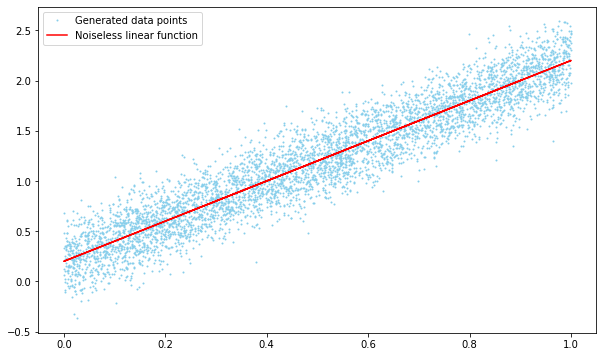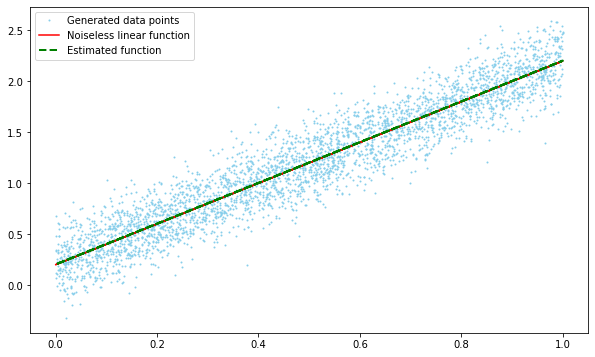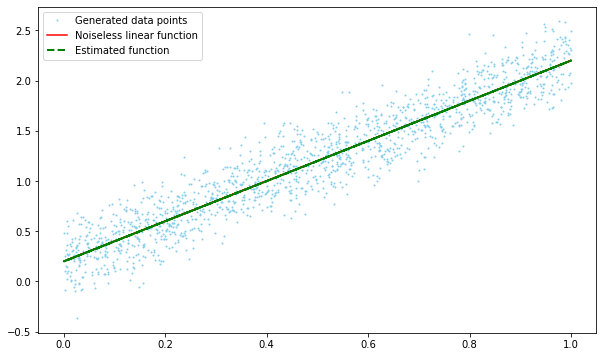# Hands-On: Linear Regression with SparkML¶

### AI and Predictive Analytics in Data-Center Environments - http://dcai.bsc.es¶

In this tutorial we will walk you through the process of using Spark ML to fit a simple Linear Regression model on some generated data. We will also introduce to the concept of DataFrame, a table-like distributed structure which serves as Spark ML's workhorse for data storage and manipulation. You will learn how to load data into a DataFrame and how to transform it into the appropriate format for the Linear Regression model.
Before doing any of that we will need some data to work with. Let's start by importing the required packages to generate the data and setting a seed for the RNG:

In :
import numpy as np
import pandas as pd
import matplotlib.pyplot as plt
# Make the figures larger
plt.rcParams['figure.figsize'] = [10, 6]

seed = 2019
np.random.seed(seed)


## Generating the data¶

For this first example we want to focus on the process of preparing the data and defining, training and testing a linear model, so we will keep the frills on the data to a minimum. Start by generating a set of points with a pretty obvious linear relationship by adding gaussian noise to a linear function and storing them in a Pandas DataFrame to ease manipulation:

In :
# Generate the dataset
y0 = 0.2
m = 2
noise_var = 0.2
num_samples = 5000
x = np.random.uniform(0, 1, num_samples)
y = y0 + m*x + np.random.normal(0, noise_var, num_samples)
linear_data_df = pd.DataFrame(data=np.column_stack([x,y]), columns=["x", "y"])

Out:
x y
0 0.903482 2.328978
1 0.393081 0.872098
2 0.623970 1.710018
3 0.637877 1.752299
4 0.880499 2.334894

Let's plot it to see what it looks like:

In :
plt.plot(linear_data_df["x"], linear_data_df["y"], 'o', markersize=1, label="Generated data points", color="skyblue");
plt.plot(linear_data_df["x"], y0 + m*linear_data_df["x"], 'r-', label="Noiseless linear function");
plt.legend();## Creating a Spark Session¶

If the notebook you are working with is not connected to a running Spark cluster you might need to create a Spark session. You can do so by typing the following:

In :
from pyspark.sql import SparkSession
spark = SparkSession.builder.master("local[*]").getOrCreate()


## Converting the data to Spark ML's DataFrame¶

Now that we have some data to play with we need to put it into a DataFrame before trying to use it to train any model. A DataFrame is Spark ML's equivalent to a table in a relational database or a a data frame in R or Python (Pandas) and it is its preferred way to store, transform and move data around. You can create a DataFrame from a Pandas Dataframe using the spark.createDataFrame() method:

In :
data_df = spark.createDataFrame(data=linear_data_df, schema=list(linear_data_df))


Notice that we pass to spark.createDataFrame() both the data as a DataFrame and the DataFrame's column names (Referred to in Spark SQL lingo as Schema, similar to relational database tables). This method is just one of many possible ways to create a DataFrame, we invite you to check the Spark ML documentation to find out which one suits your needs best.
Once the Dataframe has been created, we can print the schema and the N first rows of the DataFrame with printSchema() and show(N) respectively (show() will try to print the whole dataset if we don't indicate N):

In :
data_df.printSchema()
data_df.show(5)

root
|-- x: double (nullable = true)
|-- y: double (nullable = true)

+------------------+------------------+
|                 x|                 y|
+------------------+------------------+
|0.9034822144192743|2.3289777480698683|
|0.3930805066502425|0.8720975354883265|
|0.6239699612977534|1.7100179226174672|
|0.6378774010222266|1.7522986132110216|
|0.8804990687782621|2.3348939837531395|
+------------------+------------------+
only showing top 5 rows



DataFrames can also be easily be converted back to Pandas' with the toPandas() method:

In :
data_df.toPandas().head(5)

Out:
x y
0 0.903482 2.328978
1 0.393081 0.872098
2 0.623970 1.710018
3 0.637877 1.752299
4 0.880499 2.334894

## Generating the Linear Regression Model¶

The data is now safely stored inside a DataFrame, so we can can move on to defining the Linear Regression model:

In :
from pyspark.ml.regression import LinearRegression
lr = LinearRegression()


The LinearRegression() constructor can take multiple values that allow to fine-tune the model's behaviour, but for simplicity's sake we will provide none in this first example (because the data is generated and fairly well-posed, the model will train just fine). The 'lr' object only contains the description of the generated model, in order to be able to use it (eg. make any prediction with it) we need to first train it with data. In Spark ML models are trained through the fit() method, which takes the training data as a parameter and returns a trained model (this allows to train multiple models by providing different batches of training data to the same factory function).

In order to work properly the fit() method requires the training data to be in a specific format, namely a features column containing an array with all features for each observation and a label column containing the corresponding label. We can generate the features column by using a VectorAssembler to combine any desired amount of DataFrame columns into a column of vectors:

In :
from pyspark.ml.feature import VectorAssembler

vectorAssembler = VectorAssembler(inputCols = ['x'], outputCol = 'features')
vdata_df = vectorAssembler.transform(data_df)
vdata_df.show(5)

+------------------+------------------+--------------------+
|                 x|                 y|            features|
+------------------+------------------+--------------------+
|0.9034822144192743|2.3289777480698683|[0.9034822144192743]|
|0.3930805066502425|0.8720975354883265|[0.3930805066502425]|
|0.6239699612977534|1.7100179226174672|[0.6239699612977534]|
|0.6378774010222266|1.7522986132110216|[0.6378774010222266]|
|0.8804990687782621|2.3348939837531395|[0.8804990687782621]|
+------------------+------------------+--------------------+
only showing top 5 rows



The VectorAssembler has created a new column of vectors, each vector containing a value of x as. Although this might seem quite trivial (it simply turned a column of scalars into a column of one element vectors), it becomes quite useful once there is a large amount of columns that you want to assemble into a single column of vectors. Now that the features column has been created there's no reason for column x to stick around, so let's get rid of it. We can do so and change the name of column y to the required name label with selectExpr():

In :
prepped_df = vdata_df.selectExpr("features", "y as label")
prepped_df.printSchema()

root
|-- features: vector (nullable = true)
|-- label: double (nullable = true)



selectExpr() allows to select columns and perform SQL expressions on them, and it can be used to select features and y and change the latter's name to labels in just one function. The DataFrame is now in the appropriate format, but before training our model let's split it into training and test sets so we can validate the model later on:

In :
train_test_rate = 0.7
train_df, test_df = prepped_df.randomSplit([train_test_rate, 1-train_test_rate], seed)
print("Train rows: {}".format(train_df.count()))
print("Test rows: {}".format(test_df.count()))

Train rows: 3509
Test rows: 1491


## Training the model¶

We are now finally able to train the model:

In :
lr_model = lr.fit(train_df)
print("Coefficients: {}".format(lr_model.coefficients))
print("Intercept: {}".format(lr_model.intercept))

Coefficients: [2.0008898119693033]
Intercept: 0.20271051465436668


You have successfully trained your linear regression model! We have also printed the coefficients and the intercept value for the linear regression. If you compare it to the linear function used to generate the data ( intercept 2, coefficients [0.2] ) you can see that it did a pretty good job at estimating it!

In :
# Summarize the model over the training set and print out some metrics
trainingSummary = lr_model.summary
print("RMSE: {}".format(trainingSummary.rootMeanSquaredError))
print("r2: {}".format(trainingSummary.r2))

RMSE: 0.19857922107905726
r2: 0.8934454592988958


As expected, the RMSE corresponds almost exactly to the noise variance used to generate the data.
Plotting the original data and generating function together with the modelled line:

In :
# Because the split was done inside a dataframe we need to convert it back to a plot-friendly format
train_pd = train_df.toPandas()
x_train = np.array([x for x in train_pd['features']])
y_train = train_pd['label']
plt.plot(x_train, y_train, 'o', markersize=1, label="Generated data points", color="skyblue");
plt.plot(x_train, y0 + m*x_train, 'r-', label="Noiseless linear function");
plt.plot(x_train, lr_model.intercept + lr_model.coefficients*x_train, 'g--', linewidth=2, label="Estimated function");
plt.legend();We can see how the estimated line overlaps with the noiseless one, indicating that the model was able indeed to fit the generating function regardless of the noise.

## Testing the model¶

In order to test the model we need to use the test data. For that purpose you need to use the DataFrame's transform method, which allows to apply a transformation (eg. a model) to a given DataFrame:

In :
lr_predictions = lr_model.transform(test_df)
lr_predictions.select("prediction","label","features").show(5)

+-------------------+--------------------+--------------------+
|         prediction|               label|            features|
+-------------------+--------------------+--------------------+
| 0.2098446040207897| 0.36173475531692645|[0.00566040702338...|
| 0.2101989893818665|  0.5974935870984734|[0.00583752758798...|
|0.22676972339460036|  0.3544784944615313|[0.01411952251948...|
| 0.2285460412539866|0.018246625856927295|[0.01500731997594...|
|0.22934736853000728| 0.09392341924410447|[0.01540782054721...|
+-------------------+--------------------+--------------------+
only showing top 5 rows



From those first five predictions it doesn't look that accurate, but recall that the noise variance has a value of 0.2. You can use a Regression Evaluator to better check how good of a fit the regression was:

In :
from pyspark.ml.evaluation import RegressionEvaluator
lr_evaluator = RegressionEvaluator(predictionCol="prediction", labelCol="label",metricName="r2")
print("R Squared (R2) on test data = {}".format(lr_evaluator.evaluate(lr_predictions)))

R Squared (R2) on test data = 0.8957772537042555


The R Squared value is effectively the same as with the training data, so it seems this is indeed the best that we can do.
Plotting the modeled function against the test data:

In :
test_pd = test_df.toPandas()
x_test = np.array([x for x in test_pd['features']])
y_test = test_pd['label']
plt.plot(x_test, y_test, 'o', markersize=1, label="Generated data points", color = "skyblue");
plt.plot(x_test, y0 + m*x_test, 'r-', label="Noiseless linear function");
plt.plot(x_test, lr_model.intercept + lr_model.coefficients*x_test, 'g--', linewidth=2, label="Estimated function");
plt.legend();Again we can see that it overlaps with the function used to generate the data